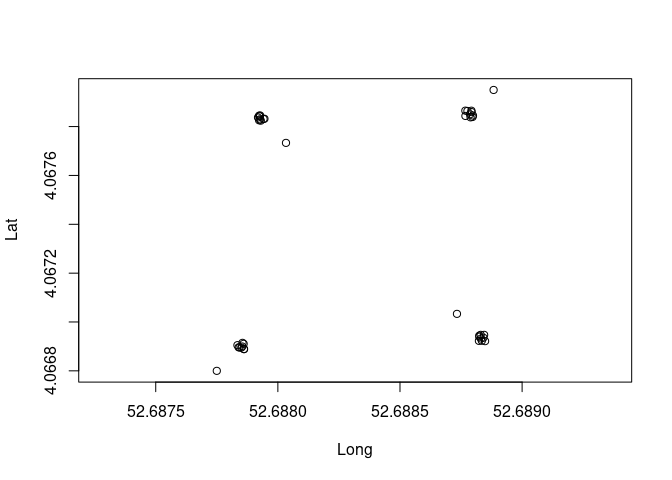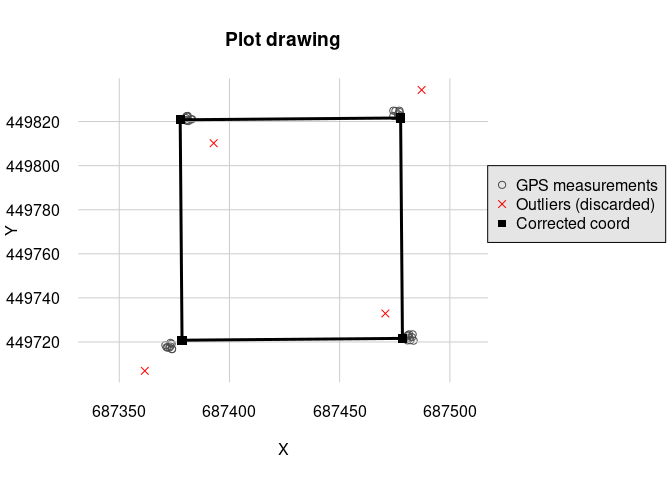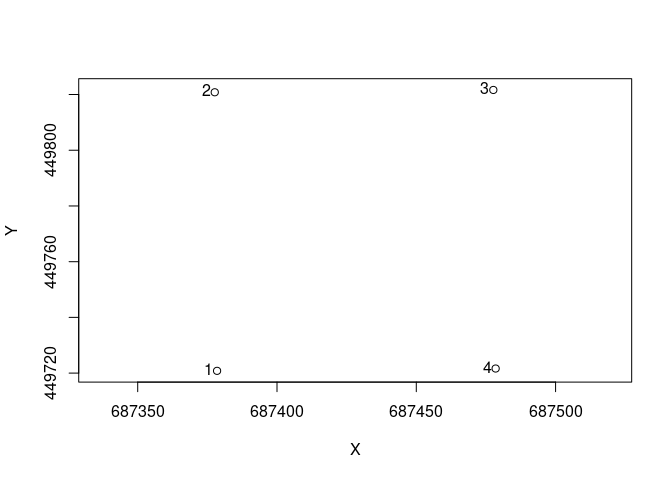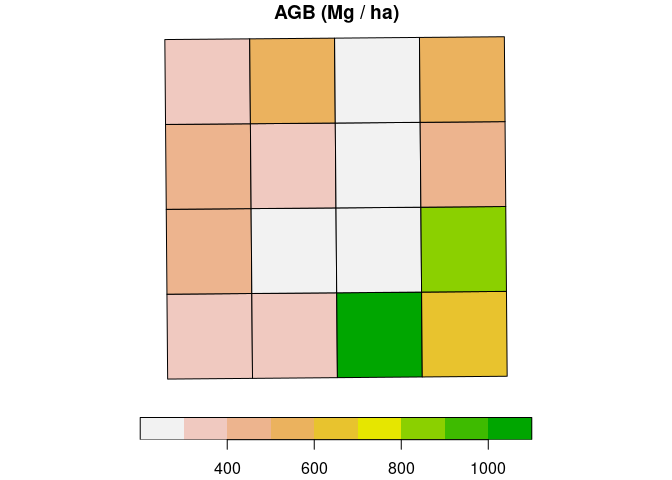# 1 Requirement

You need the following information to use the following function in your analysis :

• Dimension of the plots
• Coordinate GPS of minimum 3 points non-aligned and relatily far away with the corresponding relative coordinates
• The origin corner
• The X, Y directions
trees <- read.csv(system.file("external", "NouraguesPlot.csv",
package = "BIOMASS", mustWork = TRUE
))
plot xRel yRel D WD H
NB1 1.30 4.7 11.459 0.643 12
NB1 2.65 4.3 11.618 0.580 16
NB1 4.20 6.9 83.875 0.591 40
NB1 5.90 4.7 14.961 0.568 18
NB1 6.40 4.1 36.765 0.530 27
NB1 13.50 2.3 13.528 0.409 20

We can see in the table that we have for each trees the name of the plot we have, the xRel and yRel, the relative coordinate of the trees inside the plot. The rest of the column is for calculate the AGB at the end.

coord <- read.csv(system.file("external", "Coord.csv",
package = "BIOMASS", mustWork = TRUE
))

plot(coord[, c("Long", "Lat")], asp = 1)Plot the coordinate long lat

We can see on the plot that the corner coordinates are spread.

Plot Corners Lat Long xRel yRel
NB1 NB1_SW 4.067 52.689 0 0
NB1 NB1_SW 4.067 52.689 0 0
NB1 NB1_SW 4.067 52.689 0 0
NB1 NB1_SW 4.067 52.689 0 0
NB1 NB1_SW 4.067 52.689 0 0
NB1 NB1_SW 4.067 52.689 0 0

In the table, we have the name of the plot, the coordinate Lat, Long (or another projected coordinates), and xRel, yRel, the relative coordinate for the points observed.

# 2 To manage the plots

## 2.1 Import the dataset and visualisation

The plot is referenced in the longitude latitude coordinate so you must have the package proj4 if you are in this situation. If you have projected coordinate, you can continue with the projCoord argument instead of longlat argument.

## 2.2 Correct the GPS coordinate

correct_plot <- correctCoordGPS(
longlat = coord[, c("Long", "Lat")],
coordRel = coord[, c("xRel", "yRel")],
rangeX = c(0, 100), rangeY = c(0, 100), drawPlot = TRUE,
maxDist = 10, rmOutliers = TRUE
)
#> Le chargement a nécessité le package : proj4str(correct_plot, max.level = 1)
#> List of 5
#>  $cornerCoords :'data.frame': 4 obs. of 2 variables: #>$ correctedCoord:'data.frame':  40 obs. of  2 variables:
#>  $polygon :Formal class 'SpatialPolygons' [package "sp"] with 4 slots #>$ outliers      : int [1:4] 10 20 30 40
#>  $codeUTM : chr "+proj=utm +zone=39 +north +ellps=WGS84 +datum=WGS84 +units=m +no_defs" The output of the function is a list with a data.frame corner it’s the corner of the plot, polygon the spatial polygon, outliers the vector with the line number of the outliers and codeUTM the UTM code for the polygon. The outliers are calculated by a measure of distance between the predicted points and the GPS points. If this distance is higher than the value of maxDist, the point is considered like outliers. ## 2.3 Numbering the corner We have to number the corner of the plot, it is working if we have exactly 4 points for each plot, so we have to do the correctCoordGPS before if we have not the correct number of points. coord_num <- numberCorner( projCoord = correct_plot$cornerCoords,
plot = rep("NB1", 4),
origin = c(F, F, F, T),
clockWise = TRUE
)

plot(coord_num[, c("X", "Y")], asp = 1)
text(coord_num[, c("X", "Y")], labels = coord_num[, "corner"], pos = 2, offset = 0.2)On the graph, you can noted than the corner number 1 the origin of the plot.

## 2.4 Cut the plot in multiple subplot

subplot <- cutPlot(
projCoord = coord_num[, c("X", "Y")],
plot = coord_num[, c("plot")],
corner = coord_num[, c("corner")],
gridsize = 25, dimX = 100, dimY = 100
)
plot subplot XRel YRel XAbs YAbs corner
NB1 NB1_0_0 0 0 687378.5 449720.8 1
NB1 NB1_0_0 25 0 687378.3 449745.8 2
NB1 NB1_0_0 0 25 687403.5 449721.0 4
NB1 NB1_0_0 25 25 687403.3 449746.0 3
NB1 NB1_1_0 25 0 687378.3 449745.8 1
NB1 NB1_1_0 50 0 687378.1 449770.8 2

# 3 Trees managements

trees$subplot <- attributeTree(trees[, c("xRel", "yRel")], rep("NB1", nrow(trees)), subplot) ## 3.2 Calculate the AGB and spatialisation trees$AGB <- computeAGB(trees$D, trees$WD, H = trees$H) AGB <- summaryByPlot(trees$AGB, trees$subplot, drawPlot = TRUE, subplot = subplot)print(AGB) #>$AGB
#>       plot      AGB
#> 1  NB1_0_0 19.38352
#> 2  NB1_0_1 23.20641
#> 3  NB1_0_2 67.08190
#> 4  NB1_0_3 39.52547
#> 5  NB1_1_0 25.89987
#> 6  NB1_1_1 17.65023
#> 7  NB1_1_2 14.69783
#> 8  NB1_1_3 54.67545
#> 9  NB1_2_0 29.60386
#> 10 NB1_2_1 20.95844
#> 11 NB1_2_2 17.11330
#> 12 NB1_2_3 26.44309
#> 13 NB1_3_0 23.24261
#> 14 NB1_3_1 34.46043
#> 15 NB1_3_2 16.31269
#> 16 NB1_3_3 33.33348
#>
#> $polygon #> Simple feature collection with 16 features and 2 fields #> Geometry type: POLYGON #> Dimension: XY #> Bounding box: xmin: 687377.7 ymin: 449720.8 xmax: 687478.5 ymax: 449821.6 #> CRS: NA #> First 10 features: #> plot AGB polygon #> 1 NB1_0_0 310.1364 POLYGON ((687378.5 449720.8... #> 2 NB1_1_0 414.3979 POLYGON ((687378.3 449745.8... #> 3 NB1_2_0 473.6617 POLYGON ((687378.1 449770.8... #> 4 NB1_3_0 371.8818 POLYGON ((687377.9 449795.8... #> 5 NB1_0_1 371.3025 POLYGON ((687403.5 449721, ... #> 6 NB1_1_1 282.4038 POLYGON ((687403.3 449746, ... #> 7 NB1_2_1 335.3350 POLYGON ((687403.1 449771, ... #> 8 NB1_3_1 551.3669 POLYGON ((687402.9 449796, ... #> 9 NB1_0_2 1073.3104 POLYGON ((687428.5 449721.2... #> 10 NB1_1_2 235.1652 POLYGON ((687428.3 449746.2... ## 3.3 Attribute the trees to GPS coordinates There is two maners to attribute the trees to GPS coordinates TreeCoord <- attributeTreeCoord( xy = trees[, c("xRel", "yRel")], plot = trees$plot,
coordAbs = subplot,
dim = c(100, 100)
)
Xproj Yproj
687383.2 449722.1
687382.8 449723.5
687385.4 449725.1
687383.1 449726.7
687382.5 449727.2
687380.7 449734.3

If you want to have in GPS (longitude/latitude) coordinates (need to install proj4 first) :

#TreeCoord <- as.data.frame( proj4::project(TreeCoord, proj = correct_plot$codeUTM, inverse = TRUE) ) Head of the table TreeCoord Xproj Yproj 687383.2 449722.1 687382.8 449723.5 687385.4 449725.1 687383.1 449726.7 687382.5 449727.2 687380.7 449734.3 If you want to have the GPS (longitude/latitude) coordinates without passing through all this step however you must use the numberCorner function: coordAbs = data.frame(X = c(4.066923, 4.067865, 4.067842, 4.066905), Y = c(52.68883, 52.68877, 52.68793, 52.68783)) ncoordAbs = numberCorner(projCoord = coordAbs, plot = rep("NB1", 4), origin = c(TRUE, FALSE, FALSE, FALSE), clockWise = TRUE) TreeCoord <- attributeTreeCoord( xy = trees[, c("xRel", "yRel")], plot = trees$plot,
coordAbs = ncoordAbs,
dim = c(100, 100)
)